### Become an OU studentStart this free course now. Just create an account and sign in. Enrol and complete the course for a free statement of participation or digital badge if available.

# 3.3.2 Comparisons with other rates of return

## Inflation

In all but seriously distressed economies, inflation always occurs. £1 now is worth less than £1 in the year 2000. Similarly £1 is worth more now than £1 will be in 2025. Inflation puts a minimum figure on what the discount rate can be, because at the very worst, we want to earn a financial return from a project that is greater than the rate of inflation. A financial return lower than the rate of inflation is effectively a negative return in terms of value – the money you get back has lost value compared with the value of money invested.

It might help you to think of the discount rate as a kind of ‘inflation-plus’. As discussed above, inflation decreases the value of money over time: £1 in the future will buy you less than £1 now. However, other reasons for the time value of money also decrease the value of money over time – cash flows in the future that are more risky, for example, are worth less than cash flows that are certain or known with greater confidence. So the discount rate needs to be raised above the rate of inflation to account for the loss of value over time from inflation, and also from other factors.

## The opportunity cost of alternative investments (such as gilts, bonds, and shares)

This follows on from the loss of flexibility. Imagine that the flexibility to invest the cash in another project somewhere else is not just an abstract benefit: you know about other investments and what the return from them would be. For example, cash can be used in less risky investments such as gilts, bonds and deposit accounts (i.e. savings accounts for organisations). If investing in gilts (which are normally considered risk free) would earn 5% per year, it would be foolish to invest in any project which had a return of less than 5% per year, as this return could be achieved without any of the risk that the project involves simply by investing in gilts.

The risk from a project is usually higher than investments such as gilts or bonds, so clearly, it doesn’t make sense to invest money in a project which provides a lower return despite having a higher risk. So in the example above, the minimum value for the time value of money would be 5% per year, as that is a risk-free alternative use of the cash compared to investing it in a project. When we set a discount rate to appraise a particular project, what we are implicitly saying is that by investing in a typical project of the same risk, we could earn a return equal to the discount rate. Therefore, if the project under consideration does not produce a return of at least this discount rate, then the project is not providing a sufficient return to compensate for the risk taken, and is not worth investing in.

## How the discount factor is calculated, and how it relates to other rates of return

Though discount factors are provided to you in the Appendix, you can calculate the discount factor applicable to cash flows in any year with a simple formula. Understanding this formula may also help you to understand how discounting works. The formula for the discount factor is: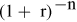Equation 2

Where r equals the discount rate as a percentage figure, e.g. 5%, and n equals the number of years into the future when the cash flow takes place. This formula is equal to: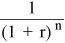Equation 3

Using some numbers for an example now, let’s say the discount rate is 5%; 5% expressed as a decimal is 0.05. The discount factor for a cash flow in year 1 would be: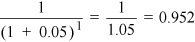Equation 4

The discount factor for a cash flow in year 3 would be: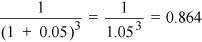Equation 5

Now, what is the logic behind this? Imagine that a gilt returns 5% per year, and we base the time value of money entirely on this alternative investment. After one year, £100 invested in the gilt will be worth 5% more, so will be £105. A project which returns more than £105 for £100 invested at the present time will be a better use of that money than investing it in the gilt (if the risk was the same), whereas a project which returns less than £105 is not a better use, and the money is better invested in the gilt.

Think about trying to do the reverse now, valuing an expected cash flow in year 1, compared to what is invested at the present time (also known as year 0). We already know that £105 in year 1 has the same value as £100 in year 0. Applying the discount factor for year 1 to a cash flow in year 1 transforms it into year 0 pounds. Therefore: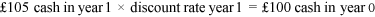Equation 6

With some simple algebra: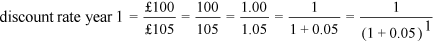Equation 7

This result is now the same as the formula for the discount factor, given above, when n equals 1 and r equals 5%. The discount factor compares a cash flow in a future year with the amount that would have been needed to be invested in year 0 at the minimum return, represented by the discount rate (in this example 5%) which would have resulted in a future cash flow of the same size as the one being discounted. Future cash flows are being discounted at the rate of the minimum return to give their equivalent amount invested in year 0.

The additional factor that makes discounting a difficult concept is that the discount rate represents more than just the minimum return of an alternative investment (5% in the example above). The discount rate also represents the risk that a project involves, and for this reason is higher than a risk-free alternative investment. However, it is possible to think of this component as the minimum return necessary to compensate an investor for the additional risk the project carries. If a hypothetical alternative investment was not risk-free, but had the same risk as the project being considered, the return which would be necessary to interest investors in that investment is equal to the discount rate that is used for the project appraisal.